﻿﻿ Harmonic Analysis of Standing Barsexamples｜products｜Murata Software Co., Ltd.# Example16Harmonic Analysis of Standing Bars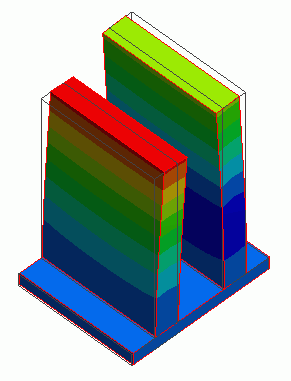General

• Forced oscillation is applied on two standing bars.

• The resulting vibrations are analyzed.

• Unless specified in the list below, the default conditions will be applied.

### Analysis Space

 Item Settings Analysis Space 3D Model unit mm

### Analysis Conditions

The analysis type is the harmonic analysis.

 Item Settings Solvers Mechanical Stress Analysis [Galileo] Analysis Type Harmonic analysis Options N/A

The resonant frequencies of each tower are 1250[Hz] and 1650[Hz], which are acquired by individual analyses.

Therefore, the sweep range is around those frequencies.

The harmonic analysis is set as follows.

 Tab Setting Item Settings Harmonic analysis Frequency Minimum: 1000[Hz] Maximum: 2000[Hz] Step Linear step: Division number: 20

### Model

Two bars (TOWER) are standing on a plate (GROUND).

The boundary condition is set on the face topology of GROUND to force the displacement in the Y direction. It is named VIBE_Y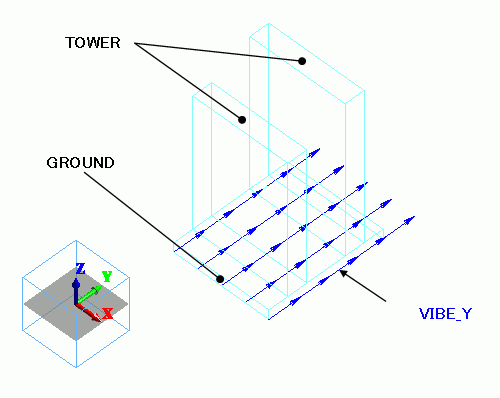### Body Attributes and Materials

 Body Number/Type Body Attribute Name Material Name 0/Solid TOWER 001_Al * 1/Solid TOWER 001_Al * 2/Solid GROUND 001_Al *

* Available from the Material DB

### Boundary Conditions

The forced displacement is applied on on the face of GROUND in the Y direction. The displacement in X and Z directions are fixed.

 Boundary Condition Name/Topology Tab Boundary Condition Type Settings VIBE_Y/Face Mechanical Displacement Select all X/Y/Z components. UX=UX=0.0, UY=1×10^-3[m]

This means the magnitude of Y displacement is 1[mm].

### Results

The displacement diagrams are shown below. The oscillation frequencies are 1000[Hz], 1250[Hz], 1450[Hz], 1600[Hz] and 2000[Hz] respectively.

The contour diagram shows the displacement.

##### 1000[Hz]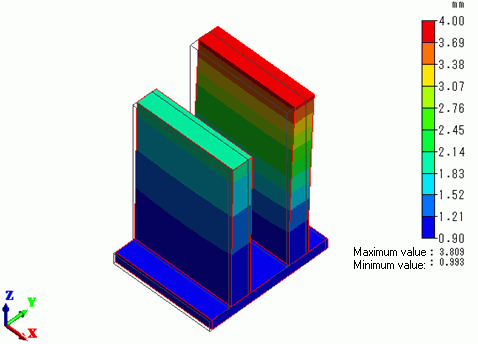##### 1250[Hz]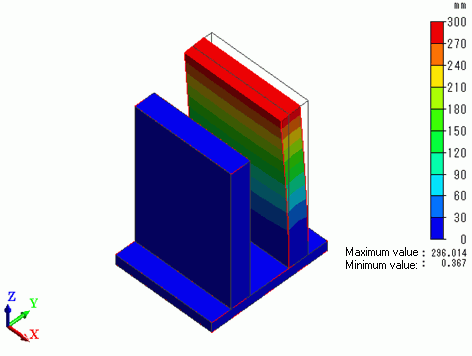##### 1450[Hz]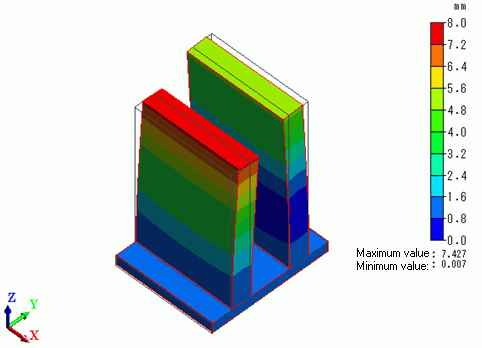##### 1600[Hz]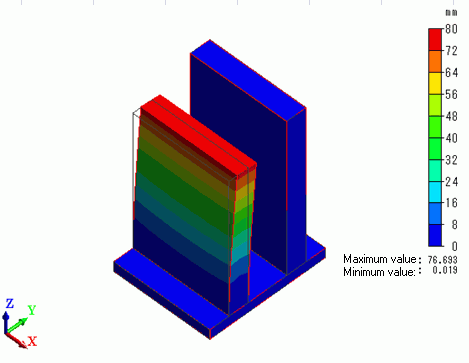##### 2000[Hz]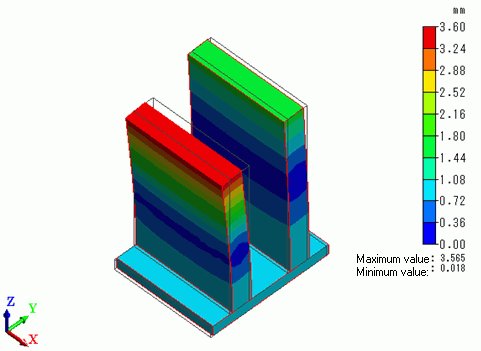The displacement is high at the resonant frequencies: 1250[Hz] and 1600[Hz]. (Watch out for the color scales)

The vibrations are out of phase for the non-resonant frequencies.

﻿ ﻿